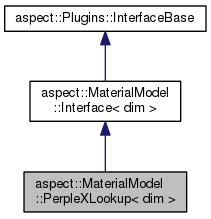ASPECT
aspect::MaterialModel::PerpleXLookup< dim > Class Template Reference
Inheritance diagram for aspect::MaterialModel::PerpleXLookup< dim >:[legend]

## Public Member Functions

void initialize () override

bool is_compressible () const override

void evaluate (const MaterialModel::MaterialModelInputs< dim > &in, MaterialModel::MaterialModelOutputs< dim > &out) const overridePublic Member Functions inherited from aspect::MaterialModel::Interface< dim >
virtual ~Interface ()=default

virtual void update ()

virtual void create_additional_named_outputs (MaterialModelOutputs &outputs) const

virtual void fill_additional_material_model_inputs (MaterialModel::MaterialModelInputs< dim > &input, const LinearAlgebra::BlockVector &solution, const FEValuesBase< dim > &fe_values, const Introspection< dim > &introspection) const

const NonlinearDependence::ModelDependenceget_model_dependence () const

## Private Attributes

std::string perplex_file_name

double eta

double k_value

double min_temperature

double max_temperature

double min_pressure

double max_pressure

## Functions used in dealing with run-time parameters

void parse_parameters (ParameterHandler &prm) override

static void declare_parameters (ParameterHandler &prm)Public Types inherited from aspect::MaterialModel::Interface< dim >
using MaterialModelInputs = MaterialModel::MaterialModelInputs< dim >

using MaterialModelOutputs = MaterialModel::MaterialModelOutputs< dim >Static Public Member Functions inherited from aspect::MaterialModel::Interface< dim >
static void declare_parameters (ParameterHandler &prm)Protected Attributes inherited from aspect::MaterialModel::Interface< dim >
NonlinearDependence::ModelDependence model_dependence

## Detailed Description

### template<int dim> class aspect::MaterialModel::PerpleXLookup< dim >

A material model that calls the thermodynamic software PerpleX in order to evaluate material properties at a given point, namely the density, heat capacity, thermal expansivity and compressibility. The viscosity and thermal conductivity are globally constant.

The usual PerpleX input files are required in the working directory: an endmember dataset file, solution model dataset file and option file. The path to a PerpleX input file must be given by the user in the ASPECT parameter file; this file may be in any directory. PerpleX output files will be written to the same directory.

Compositional fields are used to define the bulk composition at each point. These compositional fields correspond to the components as given in the PerpleX input file. The order of components is preserved.

This model requires PerpleX. A script to download and setup the required files can be found in contrib/perplex. If the default installation directory is not changed, cmake will automatically find the required files during creation of the ASPECT build files. See ./contrib/perplex/README.md

WARNING: This model is extremely slow because there are many redundant calls to evaluate the material properties; it serves only as a proof of concept.

Definition at line 63 of file perplex_lookup.h.

## § initialize()

template<int dim>
 void aspect::MaterialModel::PerpleXLookup< dim >::initialize ( )
overridevirtual

Initialization function. Loads the material data and sets up pointers.

Reimplemented from aspect::MaterialModel::Interface< dim >.

## § is_compressible()

template<int dim>
 bool aspect::MaterialModel::PerpleXLookup< dim >::is_compressible ( ) const
overridevirtual

Return whether the model is compressible or not. Incompressibility does not necessarily imply that the density is constant; rather, it may still depend on temperature or pressure. In the current context, compressibility means whether we should solve the continuity equation as $$\nabla \cdot (\rho \mathbf u)=0$$ (compressible Stokes) or as $$\nabla \cdot \mathbf{u}=0$$ (incompressible Stokes).

Implements aspect::MaterialModel::Interface< dim >.

## § evaluate()

template<int dim>
 void aspect::MaterialModel::PerpleXLookup< dim >::evaluate ( const MaterialModel::MaterialModelInputs< dim > & in, MaterialModel::MaterialModelOutputs< dim > & out ) const
overridevirtual

Function to compute the material properties in out given the inputs in in.

Implements aspect::MaterialModel::Interface< dim >.

## § declare_parameters()

template<int dim>
 static void aspect::MaterialModel::PerpleXLookup< dim >::declare_parameters ( ParameterHandler & prm )
static

Declare the parameters this class takes through input files.

## § parse_parameters()

template<int dim>
 void aspect::MaterialModel::PerpleXLookup< dim >::parse_parameters ( ParameterHandler & prm )
overridevirtual

Read the parameters this class declares from the parameter file.

Reimplemented from aspect::MaterialModel::Interface< dim >.

## § perplex_file_name

template<int dim>
 std::string aspect::MaterialModel::PerpleXLookup< dim >::perplex_file_name
private

Definition at line 101 of file perplex_lookup.h.

## § eta

template<int dim>
 double aspect::MaterialModel::PerpleXLookup< dim >::eta
private

Definition at line 102 of file perplex_lookup.h.

## § k_value

template<int dim>
 double aspect::MaterialModel::PerpleXLookup< dim >::k_value
private

Definition at line 103 of file perplex_lookup.h.

## § min_temperature

template<int dim>
 double aspect::MaterialModel::PerpleXLookup< dim >::min_temperature
private

Definition at line 104 of file perplex_lookup.h.

## § max_temperature

template<int dim>
 double aspect::MaterialModel::PerpleXLookup< dim >::max_temperature
private

Definition at line 105 of file perplex_lookup.h.

## § min_pressure

template<int dim>
 double aspect::MaterialModel::PerpleXLookup< dim >::min_pressure
private

Definition at line 106 of file perplex_lookup.h.

## § max_pressure

template<int dim>
 double aspect::MaterialModel::PerpleXLookup< dim >::max_pressure
private

Definition at line 107 of file perplex_lookup.h.

The documentation for this class was generated from the following file: